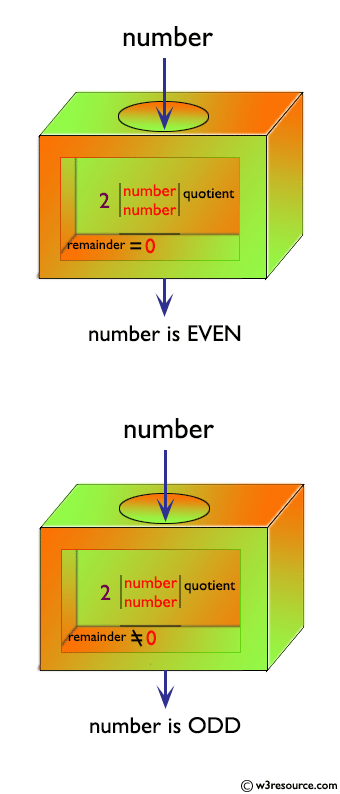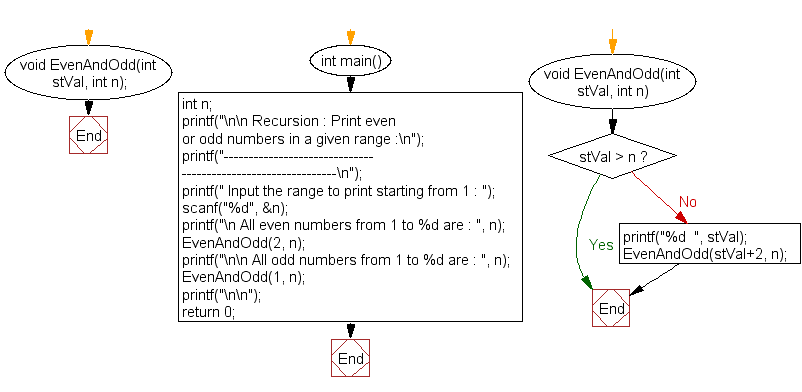﻿ C exercises: Print even or odd numbers in a given range - w3resource

# C Exercises: Print even or odd numbers in a given range

## C Recursion: Exercise-14 with Solution

Write a program in C to print even or odd numbers in a given range using recursion.

Pictorial Presentation:Sample Solution:

C Code:

``````#include <stdio.h>
void EvenAndOdd(int stVal, int n);

int main()
{
int n;
printf("\n\n Recursion : Print even or odd numbers in a given range :\n");
printf("-------------------------------------------------------------\n");

printf(" Input the range to print starting from 1 : ");
scanf("%d", &n);

printf("\n All even numbers from 1 to %d are : ", n);
EvenAndOdd(2, n);//call the function EvenAndOdd for even numbers

printf("\n\n All odd numbers from 1 to %d are : ", n);
EvenAndOdd(1, n);// call the function EvenAndOdd for odd numbers
printf("\n\n");

return 0;
}
void EvenAndOdd(int stVal, int n)
{
if(stVal > n)
return;
printf("%d  ", stVal);
EvenAndOdd(stVal+2, n);//calling the function EvenAndOdd itself recursively
}
```
```

Sample Output:

``` Recursion : Print even or odd numbers in a given range :
-------------------------------------------------------------
Input the range to print starting from 1 : 10

All even numbers from 1 to 10 are : 2  4  6  8  10

All odd numbers from 1 to 10 are : 1  3  5  7  9
```

Explanation:

```void EvenAndOdd(int stVal, int n)
{
if(stVal > n)
return;
printf("%d  ", stVal);
EvenAndOdd(stVal+2, n);//calling the function EvenAndOdd itself recursively
}
```

The function EvenAndOdd () takes two integer arguments, stVal and n, where stVal is the starting value (2 for even and 1 for odd) and n is the ending value of the range.

The function first checks if the stVal is greater than n. If it is, then the function returns without doing anything. If stVal is not greater than n, the function prints the value of stVal.

After printing the value of stVal, the function then calls itself recursively with an argument stVal+2 to print the next even/odd number in the range. This process continues until stVal becomes greater than n.

Time complexity and space complexity:

Since each call to the function only prints one value and makes one recursive call, the time complexity of this function is O(n/2), or simply O(n).

The space complexity is O(1), since the function only uses a constant amount of memory regardless of the value of n.

Flowchart:C Programming Code Editor:

Have another way to solve this solution? Contribute your code (and comments) through Disqus.

What is the difficulty level of this exercise?

Test your Programming skills with w3resource's quiz.

﻿

## C Programming: Tips of the Day

__FILE__ macro shows full path

```#include <string.h>

#define __FILENAME__ (strrchr(__FILE__, '/') ? strrchr(__FILE__, '/') + 1 : __FILE__)
For Windows use '\\' instead of '/'.
```

Ref : https://bit.ly/3iEWRoT

We are closing our Disqus commenting system for some maintenanace issues. You may write to us at reach[at]yahoo[dot]com or visit us at Facebook